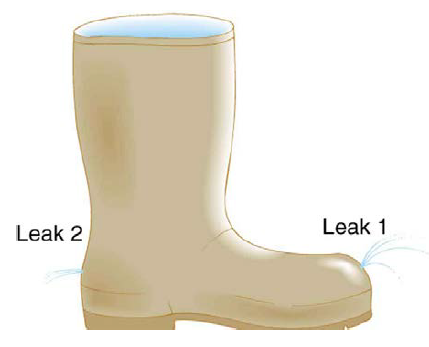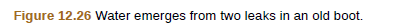Chapter 12, Problem 20CQ

Chapter
Section
Textbook Problem

The old rubber boot shown in Figure 12.26 has two leaks. To what maximum height can the water squirt from Leak 1? How does the velocity of water emerging from Leak 2 differ from that of leak 1? Explain your responses in terms of energy.To determine

The maximum height up to that the water squirt from leak 1 and the way in which the velocity of water emerging from leak 2 is differ from that of leak 1.

Explanation

Formula used:

From projectile motion, the maximum height to which the water squirt from leak 1 is,

H=u2sin2θ2g

Here,

• H is the height attained by water from the leak 1 .
• u is the velocity of water at the exit.
• θ is the angle of exit.

Calculation:

From the given figure, it is clear that the flow of water from leak 1 follows a horizontal motion.

From projectile motion, the maximum height to which the water squirt from leak 1 is,

H=u2sin2θ2g

For maximum height water should exit the leak 1 at angle of 45°.

Substitute 45° for θ in above equation

Still sussing out bartleby?

Check out a sample textbook solution.

See a sample solution

The Solution to Your Study Problems

Bartleby provides explanations to thousands of textbook problems written by our experts, many with advanced degrees!

Get Started